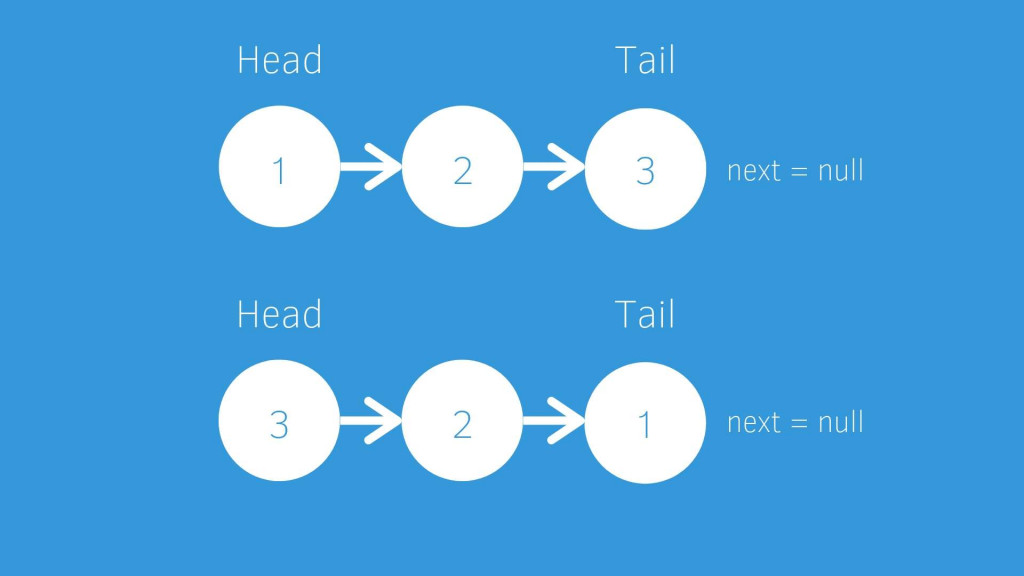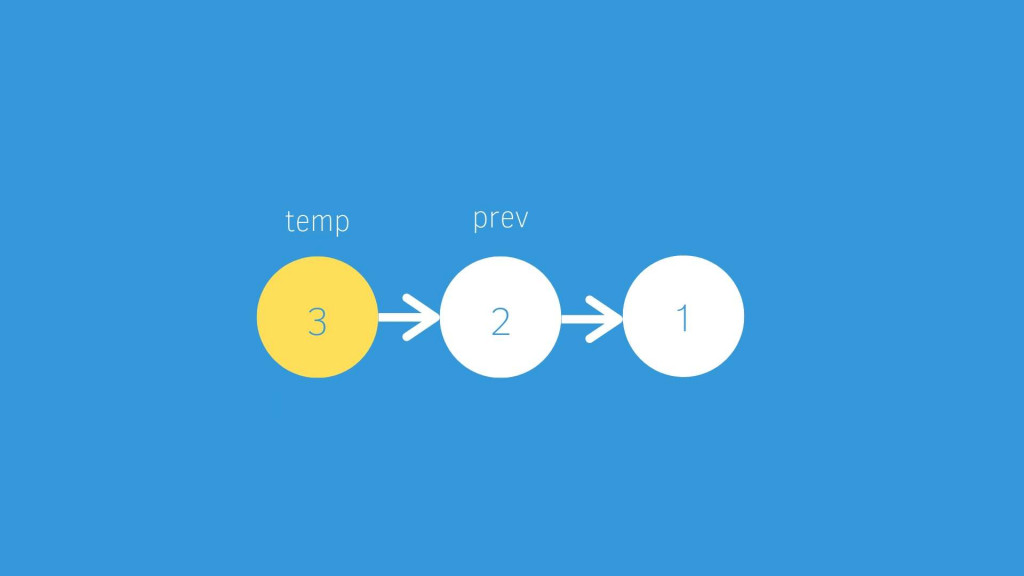#DAY 17
3
Software Development

### 前端工程師用 javaScript 學演算法系列 第 17 篇

``````// Question:
``````
``````// Example:
Input: 1->2->3->4->5->NULL
Output: 5->4->3->2->1->NULL
``````
``````/**
* function ListNode(val) {
*     this.val = val;
*     this.next = null;
* }
*/
/**
* @return {ListNode}
*/

}
``````### Think

#### 極限值/特殊狀況

• 只有一個 node (所以反轉後還是它自己)
``````// 處理極端值
return null
}
// 只有一個值所以反轉之後還是他自己
}
``````

#### 哪種資料結構解

• Linked List (範例都給你 ListNode 了)

#### 大概會怎麼解

• 首先會定義三個東西
• cur: 初始值 = head，然後判斷完會往下一個 node 移動 cur = cur.next
• temp: 暫存 cur
• prev: 初始值 = null，這個用來反轉指標
`````` let prev = null,
temp;
````````````temp = cur;   // 用 temp 來存目前的 node，不然會被後面的操作影響
cur = cur.next;   // 目前的 node 指標先往下
``````
``````// 將 next 指向先前的值
temp.next = prev;
``````
``````// prev 變成先前的值
prev = temp;
``````#### 完整程式碼

``````var reverseList = function(head) {
// 極端值
return null
}
// 只有一個值所以反轉之後還是他自己
}

let prev = null,
temp;

while(cur != null){
temp = cur;   // 用 temp 來存目前的 node，不然會被後面的操作影響
cur = cur.next;   // 目前的 node 指標先往下

// 將 next 指向先前的值
temp.next = prev;

// prev 變成先前的值
prev = temp;
}

return prev;

};

``````

``````如有錯誤或需要改進的地方，拜託跟我說。

``````